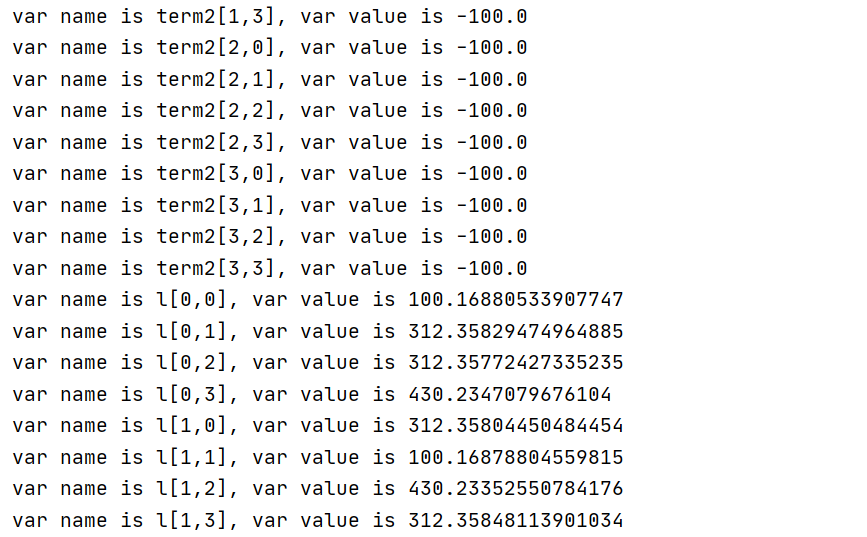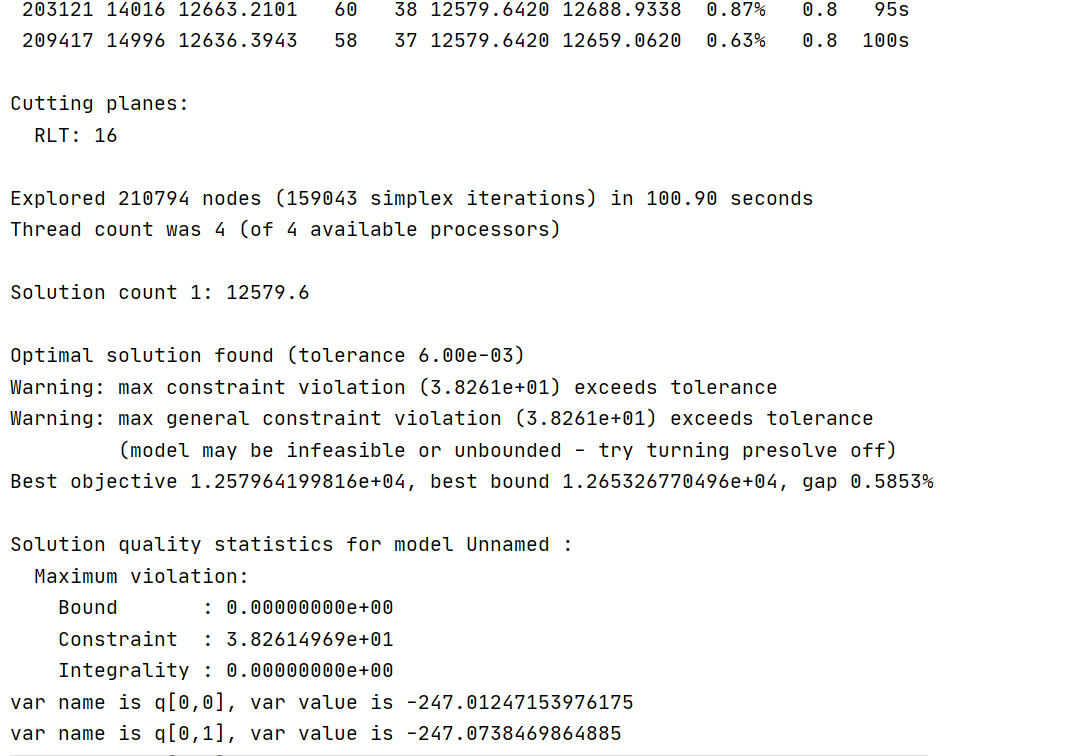•Gurobi Staff

Can you explain exactly what you are trying to do? In the code, you add new variables to the model and assign them to $$\texttt{term1}$$:

term1 = model.addVars(4, lb=-100, ub=10000, vtype=GRB.CONTINUOUS, name='term1')

Here, $$\texttt{term1}$$ is a tupledict object. $$\texttt{term1}$$ is the first variable object, $$\texttt{term1}$$ is the second variable object, and so forth. Then, you redefine every value in the $$\texttt{term1}$$ tupledict to be $$\texttt{1}$$:

for k in range(K):    term1[k] = 1

Now, $$\texttt{term1}$$, $$\texttt{term1}$$, $$\texttt{term1}$$, and $$\texttt{term1}$$ no longer correspond to the model's variables - they are all equal to the integer $$\texttt{1}$$. This has no effect on the Gurobi model or its variables. In order to make a change to a model variable, you should (e.g.) call methods of the Model or Var classes, or modify the variable object's attributes.

Are you trying to fix these variables to a certain value? If so, you should fix the lower bound and upper bound of each variable to the same value. E.g.:

for k in range(K):    val = 1    for o in range(K):        val += 1 / (np.linalg.norm(qc[o] - s[k]) ** 2)    term1[k].LB = val    term1[k].UB = val

Also, note that the optimal solution value of every $$\texttt{term1}$$ variable is $$-100$$. However, if you modify the model and re-optimize it, these variables may take on different values in the new optimal solution (unless the variables are fixed to certain values).

•Hi,

First of all, thank you very much for your reply. Because I just used gueobi, I'm sorry for the trouble I caused you.

I am optimizing a problem in which there is only one core decision variable q, In other words, other decision variables such as term1 and term2 are referenced by me to express the objective function. Although I don't know whether my method is right or not, I do so at present.

term1 is related to qc and s, where qc and s are known. In other words, term1 should be constant during optimization.

s = np.array([[-150, -150, 0], [-150, 150, 0], [150, -150, 0], [150, 150, 0]])qc = np.array([[-200, -200, 100], [-200, 200, 100], [200, -200, 100], [200, 200, 100]])for k in range(K):    term1[k] = 1    for o in range(K):        term1[k] += 1 / (np.linalg.norm(qc[o] - s[k]) ** 2)
term2 [k, j] is a formula about l [k, j], where l [k, j] is a formula about the decision variable q [k]. I don't know how to express the objective function with the decision variable q, so I introduced term2 and l. The details are as follows.
term2 = model.addVars(4, 4, lb=-100, ub=10000, vtype=GRB.CONTINUOUS, name='term2')for k in range(K):for j in range(K):    term2[k, j] = -1 * 1.44 * 10000 * (l[k, j] * l[k, j] - np.linalg.norm(qc[j] - s[k]) ** 2) / dc[k, j] ** 4
for k in range(K):    for j in range(K):        rhs = QuadExpr()        for i in range(3):            rhs += q[j, i] * q[j, i] - 2 * s[k][i] * q[j, i]        model.addConstr(l[k, j] * l[k, j] == rhs + s[k] ** 2 + s[k] ** 2 + s[k] ** 2)
Some of my questions
• In order to express my objective function, I introduce the methods of term1 and term2, right? After your explanation of term1, I find it unnecessary for me to introduce term1 as a decision variable, because term1 is not related to the decision variable q, so term1 is just a constant. However, term2 is related to the decision variable q, but the display results show that term2 is not involved in the operation. Why does the result of term2 not change with the value of q？• How can I express the objective function to make the relationship between the objective function and the decision variables smoothly? Or how to modify the relationship between term2 and decision variable q to correctly express the objective function?
The following is the overall code
def gurobi_demo():    model = grb.Model('')    qc = np.array([[-200, -200, 100], [-200, 200, 100], [200, -200, 100], [200, 200, 100]])    s = np.array([[-150, -150, 0], [-150, 150, 0], [150, -150, 0], [150, 150, 0]])    q = model.addVars(4, 3, lb=-200, ub=200, vtype=GRB.CONTINUOUS, name='q')    term1 = model.addVars(4, lb=-100, ub=10000, vtype=GRB.CONTINUOUS, name='term1')    term2 = model.addVars(4, 4, lb=-100, ub=10000, vtype=GRB.CONTINUOUS, name='term2')    dc = np.array([[0, 0, 0, 0], [0, 0, 0, 0], [0, 0, 0, 0], [0, 0, 0, 0]])    for k in range(4):        for j in range(4):            dc[k, j] = np.linalg.norm(qc[j] - s[k]) / 10    model.update()    sum = LinExpr()    for k in range(K):        term1[k] = 1        for o in range(K):            term1[k] += 1 / (np.linalg.norm(qc[o] - s[k]) ** 2)        sum += math.log(term1[k])        for j in range(K):            term2[k, j] = -1 * 1.44 * 10000 * (l[k, j] * l[k, j] - np.linalg.norm(qc[j] - s[k]) ** 2) / dc[k, j] ** 4            sum += term2[k, j] / term1[k]    model.setObjective(sum, sense=GRB.MAXIMIZE)       for k in range(K):        model.addConstr(q[k, 2] == 100)           # np.linalg.norm(q[j] - s[k])    for k in range(K):        for j in range(K):            rhs = QuadExpr()            for i in range(3):                rhs += q[j, i] * q[j, i] - 2 * s[k][i] * q[j, i]            model.addConstr(l[k, j] * l[k, j] == rhs + s[k] ** 2 + s[k] ** 2 + s[k] ** 2)    model.setParam('NonConvex', 2)    model.setParam('NumericFocus', 3)    # model.setParam('Method', 2)    # model.setParam('Presolve', 2)    # model.setParam('MIPFocus', 3)    # model.setParam('MIPGap', 0.0005)    model.optimize()    for v in model.getVars():        print('var name is {}, var value is {}'.format(v.VarName, v.X))
•Gurobi Staff

Yes, there isn't a need for $$\texttt{term1}$$ to be a model variable. Like you mentioned, the $$\texttt{term1}$$ values are constants. So you can define $$\texttt{term1}$$ as a dictionary and populate its values in your $$\texttt{for}$$ loop:

term1 = {}for k in range(K):    term1[k] = 1    for o in range(K):        term1[k] += 1 / (np.linalg.norm(qc[o] - s[k]) ** 2)

Regarding the $$\texttt{term2}$$ variables: there is a difference between Python variables and Gurobi's model variables. You initially create new model variables (named $$\texttt{term2}$$), and assign the variable objects to the $$\texttt{term2}$$ Python variable.

term2 = model.addVars(4, 4, lb=-100, ub=10000, vtype=GRB.CONTINUOUS, name='term2')

With the line of code below, you overwrite the variable objects stored in the $$\texttt{term2}$$ Python variable with new values. This statement has no effect on the model - it just modifies the $$\texttt{term2}$$ Python variable. The $$\texttt{term2}$$ model variables are still in the model, but have not been used.

term2[k, j] = -1 * 1.44 * 10000 * (l[k, j] * l[k, j] - np.linalg.norm(qc[j] - s[k]) ** 2) / dc[k, j] ** 4

If you want Gurobi to set each $$\texttt{term2}$$ model variable equal to $$- 14400 \ell_{kj}^2 - ||qc_j-s_k||^2/dc_{kj}^4$$, you should add a constraint to the model using Model.addConstr():

model.addConstr(term2[k, j] == -1 * 1.44 * 10000 * (l[k, j] * l[k, j] - np.linalg.norm(qc[j] - s[k]) ** 2) / dc[k, j] ** 4)

Hopefully, this resolves the issues you're having. You can always use Model.write() to write an LP model file to disk:

model.write('mymodel.lp')

The LP file format is human-readable, so you can inspect it to make sure your model is formulated properly.

•Hi，

Thank you for your careful reply. Now the solution information shows that term1 and term2 can participate in the operation normally. The result of term2 optimization can also correspond to the decision variable q.

In the process of solving, I seem to have encountered the problem of tolerance accuracy. The warning information is as follows.• Do I need to check the slack of all constraints to know which constraint has max constraint violation or max general constraint? I didn't find how to view the Slack or QCSlack of constraints in the API. How to write the code?
• In the iterative algorithm, if such a warning occurs in the first solution process, what will be the impact of the optimal solution obtained in the first solution as the input of the second solution process? At present, the situation I encounter is that I can't solve the second solution.

Best regards,

Yanbo

•Gurobi Staff

The name of the constraint corresponding to the maximum constraint violation should be printed in Model.printQuality(). It looks this does not happen if the most violated constraint is an SOS or general constraint. We will work on improving this for a future release.

For now, to know exactly which constraint is most violated, you could use the approach described in the documentation for ConstrVioIndex. But I'm not sure this is necessary - because the "max constraint violation" and "max general constraint violation" are equal, it's clear that one of your general constraints is violated by $$38.2614969$$. Based on your other community post, this must be a logarithmic general constraint. I see Jaromił already described how Gurobi approximates the logarithmic function with a piecewise-linear formulation (see the documentation for Function Constraints), so tightening the bounds on the $$\texttt{term4}$$ variables should yield a better approximation and address the large general constraint violations.

If you have a large constraint violation like this, the solution may not make sense. Feeding a bad solution to the next iteration of your algorithm could definitely cause problems.

To query the Slack or QCSlack attributes, store the constraint objects returned by Model.addConstr(). After optimizing, query the corresponding attribute of that constraint object. For example:

# c is a Constr objectc = model.addConstr(x + y <= 5)model.optimize()print(c.Slack)
•Hi Eli,

If you have a large constraint violation like this, the solution may not make sense. Feeding a bad solution to the next iteration of your algorithm could definitely cause problems.

• The optimal solution obtained from the previous optimization has a large constraint violation. But the result is also in the feasible solution set. Why a bad solution to the next iteration of my algorithm, the previous result is only entered as a constant in the next iteration, could definitely cause problems？
• Can some constraint violations be solved by scale the coefficients?
•Gurobi Staff
The optimal solution obtained from the previous optimization has a large constraint violation. But the result is also in the feasible solution set. Why a bad solution to the next iteration of my algorithm, the previous result is only entered as a constant in the next iteration, could definitely cause problems

The solution isn't feasible to the constraints as defined in the model. If you plug in the solution values of the $$\texttt{term4}$$ variables to $$\texttt{math.log2()}$$ and compare the results to the solution values of the corresponding $$\texttt{lv}$$ variables, they will differ by as much as $$38.2614969$$. In general, this can certainly cause problems for an iterative algorithm. However, if the $$\texttt{lv}$$ variable values are not an important part of your algorithm, perhaps it does not matter.

Can some constraint violations be solved by scale the coefficients?

•Hi Eli,

In a word, thank you very much for your patient reply. You have helped me a lot, thank you!

Best regards,

Yanbo